# H2 Math Tue 5pmMF26

Differentiate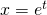with respect to t.Substituteinto given differential equation,SinceUsing the product to sum formula as shown here, we have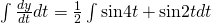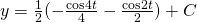Note:and— (1)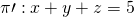— (2)— (3)
Using GC,— (1)— (2)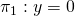— (3)
Using GC,Qn11
(i)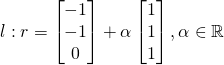(ii) Since l is the common line of intersection onand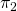, we need l to be ontoo. For that to happen,
1. l must be parallel to, that is, direction of l is perpendicular to normal to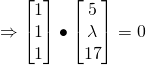2. Given that l is parallel to(since), we need l to be on, so we need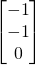to be on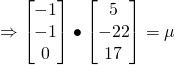(iii)
For 3 planes to have nothing in common, then l must be parallel to(Note: if l is not parallel, l will cutat a point, which means that 3 planes will cut at a point)from (ii)
But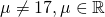12.
(i)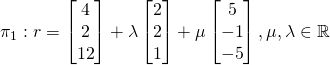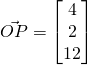is onwhenNot readable? Change text.